# Digital-to-Analog Conversion

## Digital Circuits

• #### Question 1

If a pulse-width modulated (PWM) signal is sent to a passive integrator circuit from a circuit capable of both sourcing and sinking current (as is the case with the dual-MOSFET output stage), the output will be a DC voltage (with some ripple):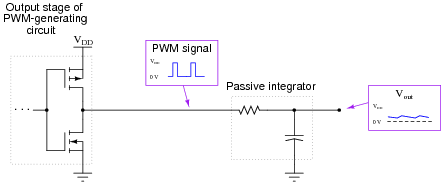Determine the relationship between the PWM signal’s duty cycle and the DC voltage output by the integrator. What does this suggest about PWM as a means of communicating information, such as analog data from a measuring device?

• #### Question 2

Explain what the purpose of a digital-to-analog converter, or DAC circuit is, in your own words.

• #### Question 3

Explain how this digital-to-analog converter (DAC) circuit is supposed to function: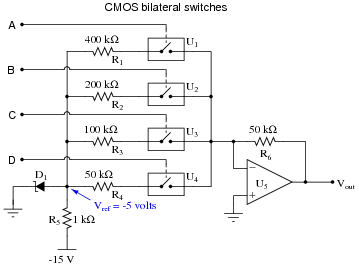• #### Question 4

A type of resistor network known as an R-2R ladder is often used in digital-to-analog conversion circuits:When all switches in the R-2R ladder are in the “ground” position, the network has a very interesting property regardless of its size. Analyze the Thévenin equivalent resistance (as seen from the output terminal) of the following R-2R ladder networks, then comment on the results you obtain:• #### Question 5

When only the most significant bit (MSB) of an R-2R ladder resistor network is activated (all other bits inactive, their switches connecting to ground), the output voltage will be the same, regardless of how many bits the network has:Explain why this output voltage magnitude stands independent of the number of bits (sections) in the R-2R ladder network.

• #### Question 6

Thévenin’s theorem is a powerful tool for analyzing R-2R ladder networks. Take for instance this four-section network where the next-to-most-significant “bit” is activated, while all the other “bits” are inactive (switched to ground):If we Thévenize all sections to the left of the activated section, replacing it with a single resistance to ground, we see the network becomes far simpler: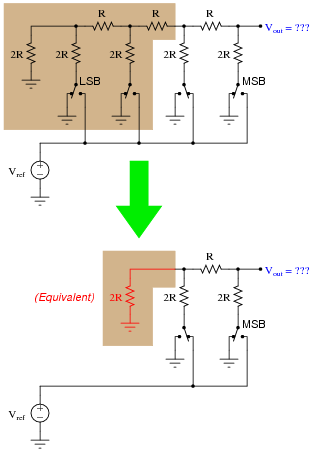Explain how we may apply Thévenin’s theorem once again to the shaded section of this next circuit (simplified from the previous circuit shown above) to simplify it even more, obtaining a final result for Vout: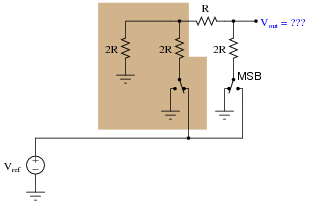• #### Question 7

Determine the voltage output by the following R-2R ladder network given the switch states shown in the table:SW0 SW1 SW2 SW3 Vout Ground Ground Ground Vref Ground Ground Vref Ground Ground Vref Ground Ground Vref Ground Ground Ground Ground Ground Ground Ground

• #### Question 8

Explain why DAC circuits based on R-2R ladder networks are more popular than binary-weighed resistor networks. Either one will work well if properly designed and built, so why would one design be more widely manufactured?

• #### Question 9

Explain what a digital potentiometer is, and give one example of a digital potentiometer in integrated-circuit (IC) form.

• #### Question 10

What is meant by the word resolution in reference to an ADC or a DAC? Why is resolution important to us, and how may it be calculated for any particular circuit knowing the number of binary bits?

• #### Question 11

This digital-to-analog converter (DAC) circuit takes a four-bit binary input (input terminals A through D) and converts it to an analog voltage (Vout). Predict how the operation of this circuit will be affected as a result of the following faults. Consider each fault independently (i.e. one at a time, no multiple faults):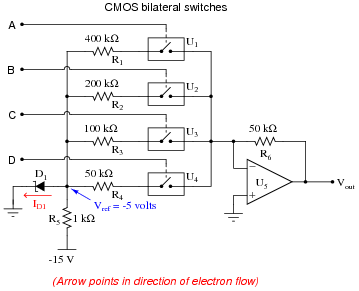Bilateral switch U1 fails open:
Zener diode fails shorted:
Solder bridge (short) past resistor R1:
Resistor R6 fails open:
• #### Question 12

The following circuit generates an analog output voltage proportional to the value of the binary input, using pulse-width modulation (PWM) as an interim format. An eight-bit binary counter (CTR) continually counts in the üp” direction, while an 8-bit magnitude comparator (CMP) checks when the 8-bit binary input value matches the counter’s output value. The AND gate and inverter simply prevent the S-R latch from being “set” and “reset” simultaneously (when both A and B are maximum, both at a hex value of \$FF), which would cause the output to be “invalid” when S and R were both active, and unpredictable when both S and R inputs returned to their inactive states:Explain how this circuit works, using timing diagrams if necessary to help show the PWM signal at $$\bar{Q}$$ for different input values.

• #### Question 13

This is a digitally-set motor speed controller circuit, using PWM to modulate power to the motor. Predict how the operation of this circuit will be affected as a result of the following faults. Consider each fault independently (i.e. one at a time, no multiple faults):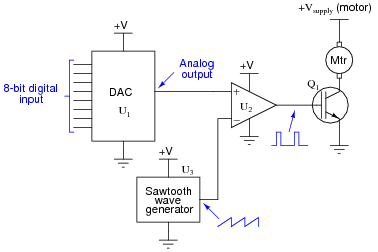DAC output fails low (output = 0 volts DC):
DAC output fails high (output = V):
IGBT Q1 fails open (collector to emitter):
Solder bridge (short) between MSB input on U1 and ground: